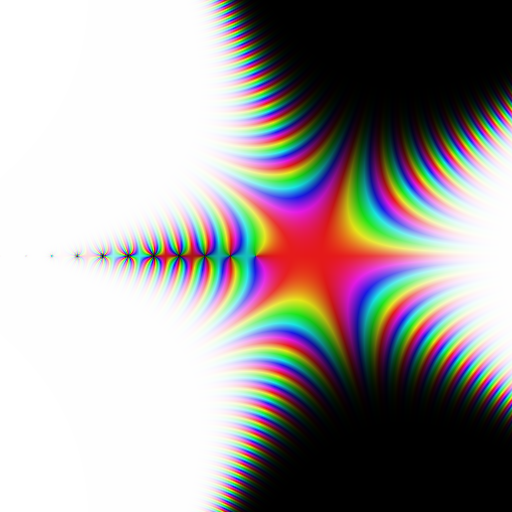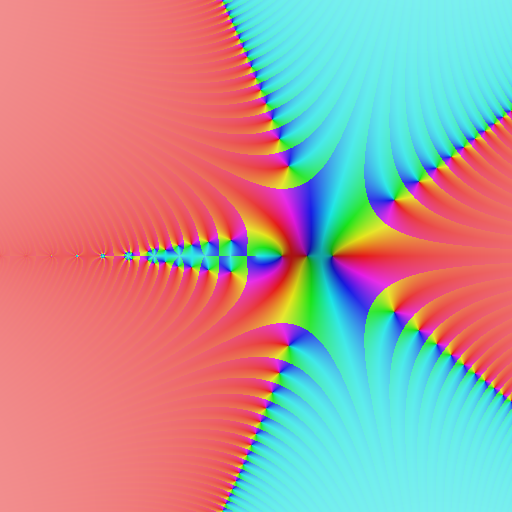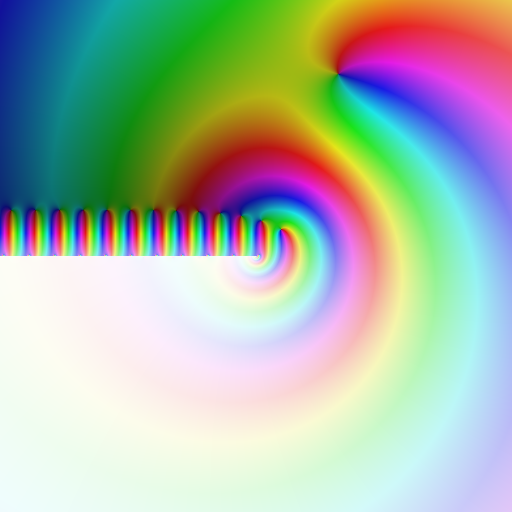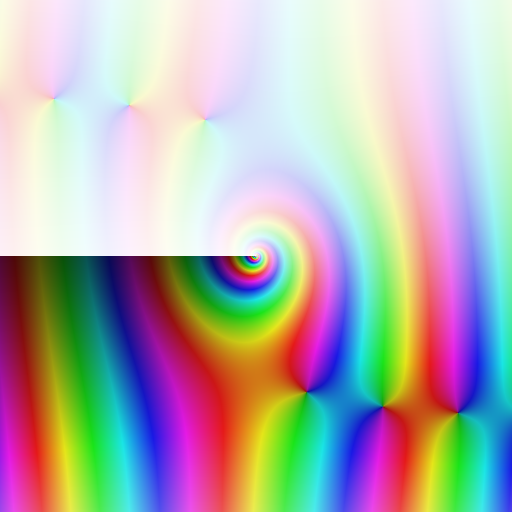# What's new in Arb 2.7.0

July 14, 2015

I've tagged version 2.7.0 of Arb. As usual, the documentation is available.

Arb 2.7.0 computes more mathematical functions and better. More below.

## Gamma-related functions

The Barnes G-function, also known as the double gamma function, has been implemented. Whereas the usual gamma function satisfies $\Gamma(z+1) = z \Gamma(z)$, the Barnes G-function satisfies $G(z+1) = \Gamma(z) G(z)$.Plot of the Barnes G-function $G(z)$ on $[-10,10] + [-10,10] i$.

The logarithmic Barnes G-function $\log G(z)$ is provided as a separate function. This is done for two reasons. Firstly, just like the logarithmic gamma function, this function is defined differently from $\log(G(z))$, to give a continuous structure in the complex plane (the branch cuts are placed on the negative half real axis). Contrast the two plots below.Left: $\log G(z)$ (correct). Right: $\log(G(z))$ (incorrect). Region: $[-10,10] + [-10,10] i$.

Secondly, it avoids huge values. For example, one has

$$G(10^{10}) = [4.83236631331771 \cdot 10^{467427913765589957090} \pm 2.41 \cdot 10^{467427913765589957075}]$$

compared to the more manageable

$$\log G(10^{10}) = [1.07629254628595 \cdot 10^{21} \pm 3.72 \cdot 10^6].$$

Either value is actually computed instantly (but the second value requires less working precision). Obviously, Arb doesn't use the recurrence relation here, which would require $10^{10}$ bignum operations; it computes the Barnes G-function and its logarithm via the Hurwitz zeta function. This also accounts for the extension of the Barnes G-function from a function on the integers to a function of a complex variable.

Another function that has been implemented based on the Hurwitz zeta function is the generalized polygamma function as defined by Espinosa and Moll, pictured below. For nonnegative integers $n$, one has $\psi(n,z) = \frac{d^{n+1}}{dz^{n+1}} \log \Gamma(z)$. The generalized polygamma function extends this function to fractional, negative and complex orders $n$ in a natural way.The generalized polygamma function $\psi(-1-4i,z)$. Region: $[-10,10] + [-10,10] i$.

A long overdue fix is that I've implemented use of the reflection formula for the logarithmic gamma function. Thus one can now instantly evaluate, say $$\log \Gamma(-10^{10} + 100i) = [-220258509624.158 \pm 1.85 \cdot 10^{-4}] + [-31415924234.8836 \pm 3.59 \cdot 10^{-5}]i$$ which previously would just have hung for a very long time. The reflection formula essentially reads $$\log \Gamma(z) = \log \Gamma(1-z) + \log(\pi) - \log \sin(\pi z)$$ but the function denoted $\log \sin(\pi z)$ must be constructed correctly, again so that one gets the expected continuous version of $\log \Gamma(z)$ with branch cuts only on the negative real half axis. The difference is illustrated below.Left: $\log \sin(\pi z)$ (correct). Right: $\log(\sin(\pi z))$ (incorrect). Region: $[-10,10] + [-10,10] i$.

I've also added support for evaluating the digamma function of a power series. One could just take the gamma function and differentiate, but having the digamma function as a standalone function is much nicer (and more efficient).

## More Bessel functions

The Bessel functions $Y_{\nu}(z)$, $I_{\nu}(z)$ have been implemented, which means that Arb now provides the full quadruple of Bessel functions (J, Y, I, K). Well, there are still many missing Bessel-related functions: spherical Bessel functions, Hankel functions, Airy functions, Bessel function zeros, and of course the Bessel functions of power series... I'd like to implement these properly some day, but if anyone desperately needs them right now, it's possible to duct tape them out of the available Bessel functions.Top row: $J_{-1-4i}(z)$, $Y_{-1-4i}(z)$. Bottom row: $I_{-1-4i}(z)$, $K_{-1-4i}(z)$. Region: $[-10,10] + [-10,10] i$.

## A bug in the Bessel K function

A severe bug has been fixed that resulted in wrong values for $K_{\nu}(z)$ with negative real $z$. For example, with $\nu = 1$ and $z = -50$, at 64-bit precision, Arb 2.6.0 would compute: $$[\pm 14.1] + [9.1202903714631487 \cdot 10^{20} \pm 5.36 \cdot 10^{3}]i$$ but at 128-bit precision, it would compute: $$[\pm 1.28 \cdot 10^{-15}] + [-912029037146314866501.125860181005431 \pm 8.55 \cdot 10^{-16}]i.$$

The first result is wrong: it is the complex conjugate of the second result, which is correct.

Yes, I found an actual bug in Arb that resulted in incorrect values (I've only had a couple of these)! How did it ever manage to slip through? The randomized unit test for the Bessel K function was designed to catch problems of this kind, by computing a value at two different levels of precision or with two different algorithms and verifying that the results are consistent. However, the number of test iterations (2000) was unfortunately set just slightly too low to trigger a failure.

This bug was revealed when testing the Bessel I and Y functions. There are interrelations between the various Bessel functions that should hold for all arguments, and the test code for the new Bessel Y function reported that this wasn't always the case. After convincing myself that the Bessel Y function actually was being computed correctly, I traced the problem back to the Bessel K function.

The problem was due to using $(\pi / (2z))^{1/2}$ as a prefactor for the asymptotic expansion of the Bessel K function. The correct prefactor is $(2z /\pi)^{-1/2}$. These expressions disagree precisely for negative real $z$.

The "incorrect" version of the prefactor is actually the one found in various reference works (the Digital Library of Mathematical Functions, for example). Strictly speaking, the "incorrect" version is correct in the sense that it just corresponds to a different branch of the Bessel K function, and you can choose any branch cut you like for a multivalued function. But at least in a software library, you surely want to choose the least surprising branch! Here, the least surprising branch choice agrees with the usual branch choice for the natural logarithm, which is continuous from above (at least, this is the convention in Arb and in all computer algebra systems I've used). Most importantly, you want the software to be consistent with itself, and picking a different branch depending on the precision is not very consistent!

## Faster summation of hypergeometric series

Two more algorithms have been added for evaluation of hypergeometric series over the complex numbers (which in turn affects Bessel functions, exponential integrals, incomplete gamma functions, and so on).

Binary splitting speeds up evaluation when all the inputs have short bit length, for example when all real and imaginary parts are small integers or $2^{-n}$ times integers (more generally one could use binary splitting for Gaussian rationals or even algebraic numbers, but there is currently no interface for passing such numbers exactly as input). The following table shows timings (in seconds) for computing the Bessel function $J_{\nu}(z)$ with $\nu = 1$, $z = \tfrac{1}{8}(7+9i)$ to a precision of D digits:

 D mpmath 0.19 Arb 2.6.0 Arb 2.7.0 100 0.00019 0.000031 0.000031 1000 0.0019 0.00041 0.00021 10000 0.37 0.017 0.0029 100000 68 1.2 0.074 1000000 103 1.1

As another point of reference, if you use the generic code for complex hypergeometric functions to evaluate the constant $e = \sum_{k=0}^{\infty} \frac{1}{k!} = {}_0F_0(1)$, it now takes 0.52 seconds to compute 1 million digits, whereas the specialized function arb_const_e takes 0.37 seconds.

Fast multipoint evaluation speeds up evaluation when the series has no special form, i.e. when all the inputs are full-precision numbers. The following timings (in seconds) are for computing the Bessel function $J_{\nu}(z)$ with $\nu = \sqrt{1 + 2i}$, $z = \sqrt{3 + 4i}$:

 D mpmath 0.19 Arb 2.6.0 Arb 2.7.0 100 0.00055 0.000089 0.000089 1000 0.27 0.0057 0.0057 10000 75 / 7.5 / 1.6 4.0 / 2.5 / 1.6 2.9 / 1.4 / 0.48 100000 1110 / 566 / 401 767 / 226 / 61

At 10000 and 100000 digits, three different timings are shown. When computing $J_{\nu}(z)$ for non-integer order $\nu$, the bottleneck becomes computing the gamma factor $\Gamma(\nu)$. The first timing is the total time for the first evaluation, which includes time to generate Bernoulli numbers for the gamma function. The second timing is for a second call to the Bessel function, with Bernoulli numbers already cached. The third timing is the time minus time to evaluate the gamma factor, i.e. for just evaluating the hypergeometric series. Clearly, a lot of time can be saved by caching the gamma factor if $\nu$ is fixed and several different $z$ values are to be evaluated (there is currently no interface for this, but it's easy to do ad-hoc if necessary for a given application).

## Elementary functions

Some more elementary functions have been added, including hyperbolic functions for complex input (acb_sinh, acb_cosh, acb_sinh_cosh, acb_tanh, acb_coth). Numerical stability of tan and cotangent functions in the complex plane has also been improved. For example, at 64-bit precision, Arb 2.6.0 gives $$\tan(1 + \tfrac{1}{3} 10^{10} i) = [1.73004383 \cdot 10^{-2895296546} \pm 7.51 \cdot 10^{-2895296555}] + [1.0000000 \pm 3.73 \cdot 10^{-9}]i$$ $$\tan(1 + \tfrac{1}{3} 10^{20} i) = [\pm \infty] + [\pm \infty]i$$ whereas Arb 2.7.0 gives $$\tan(1 + \tfrac{1}{3} 10^{10} i) = [1.73004383 \cdot 10^{-2895296546} \pm 4.28 \cdot 10^{-2895296555}] + [1.00000000000000 \pm 2 \cdot 10^{-19}]i$$ $$\tan(1 + \tfrac{1}{3} 10^{20} i) = [\pm 1.21 \cdot 10^{-28952965460216788507}] + [1.00000000000000 \pm 2 \cdot 10^{-19}]i.$$

Finally, the functions for computing n-th roots of real numbers (arb_root, arb_pow_fmpq) have been improved, removing a lot of overhead at low precision (also, code was added to use a fast algorithm when n is huge). The following table shows the time in microseconds to compute a cube root of a real number at B bits of precision:

 B MPFR 3.1.1 Arb 2.6.0 Arb 2.7.0 32 1.2 3.7 1.2 64 1.5 4.1 1.5 128 2.7 5.6 2.0 256 3.4 6.4 3.7 512 4.0 7.0 4.6 1024 5.4 9.1 5.7 2048 9.1 12.8 9.6 4096 19.0 24.3 20.2

## What's next?

There's a lot to do... I want to clean up the code for computing gamma and zeta functions and optimize it a bit. This is easy but boring work. There's much more to do for hypergeometric functions. I also want to add more support for L-functions and modular forms. More optimizations to basic arithmetic are definitely possible. And if I find the time, I should probably help out with the Sage interface to Arb that Clemens Heuberger and Marc Mezzarobba have been working on.